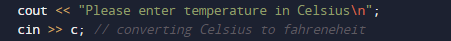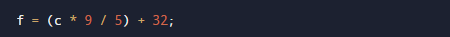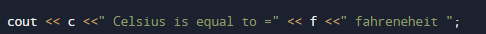# C++ Program to Convert Celsius to Fahrenheit

In this tutorial you will learn about the C++ Program to Convert Celsius to Fahrenheit and its application with practical example.

In this tutorial, we will learn to create a c++ program that Convert Celsius to Fahrenheit  using c++ programming

## Prerequisites

Before starting with this tutorial we assume that you are best aware of the following C++ programming topics:

• Operators.
• looping statements.
• Basic input/output.
• Basic c++ programming
• For Loop.
• String.

## What Is Temperature ?

Temperature is a quantity that measured the freezing and boiling point of water. Which is measured in different scales like Celsius and Fahrenheit.
In Celsius scale, the boiling point of water is 100°C, and the freezing point is at 0°C, while in the Fahrenheit scale the boiling point of water is 212°F and freezing point is at 32°F.

## C++ Program to Convert Celsius to Fahrenheit.

In this program we will convert temperature from Celsius to Fahrenheit . We would first declared and initialized the required variables. Next, we would prompt user to input the value in Celsius. Later we will Convert the values into Fahrenheit.

To convert values from Celsius  to Fahrenheit  we will use following Formula:

f = (c * 9 / 5) + 32 where, F is temperature in Fahrenheit and C is temperature in Celsius.

## Logic to find Centigrade to Fahrenheit

First of all Take the Celsius Temperature in c
Then calculate f = (c * 9 / 5) + 32.
return f.

In the above program, we have first declared and initialized a set variables required in the program.

• f = it will hold Fahrenheit value.
• c= it will hold Celsius value.

And  in the next statement user will be prompted to enter value in Celsius  and  which will be assigned to variable ‘c‘.After that with the help of  formula we will convert the value in to Fahrenheit .As you can see in above program, we first take value in Celsius  as input from user. Then convert the value of Celsius into Fahrenheit  using above conversion equation and print the Temperature in Fahrenheit .In this tutorial we have learn about the C++ Program to Convert Celsius to Fahrenheit and its application with practical example. I hope you will like this tutorial.